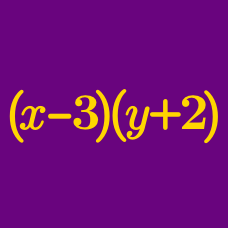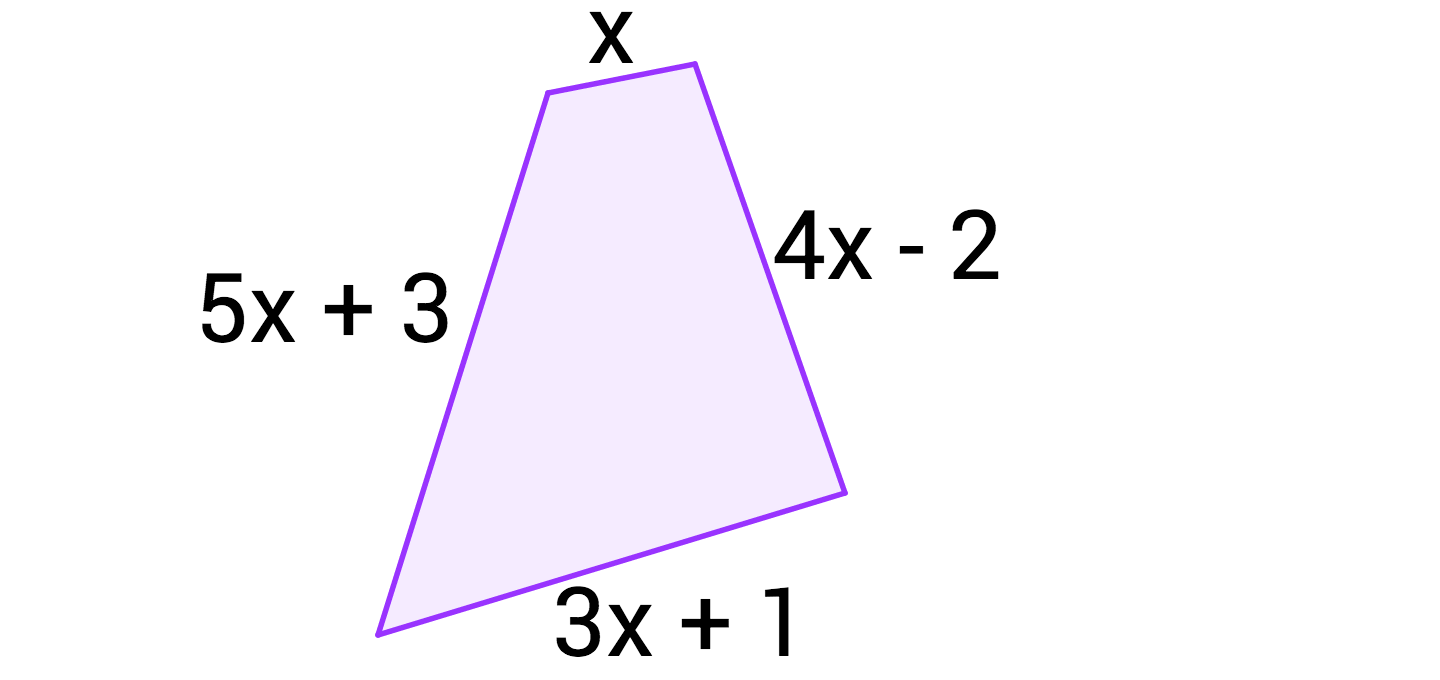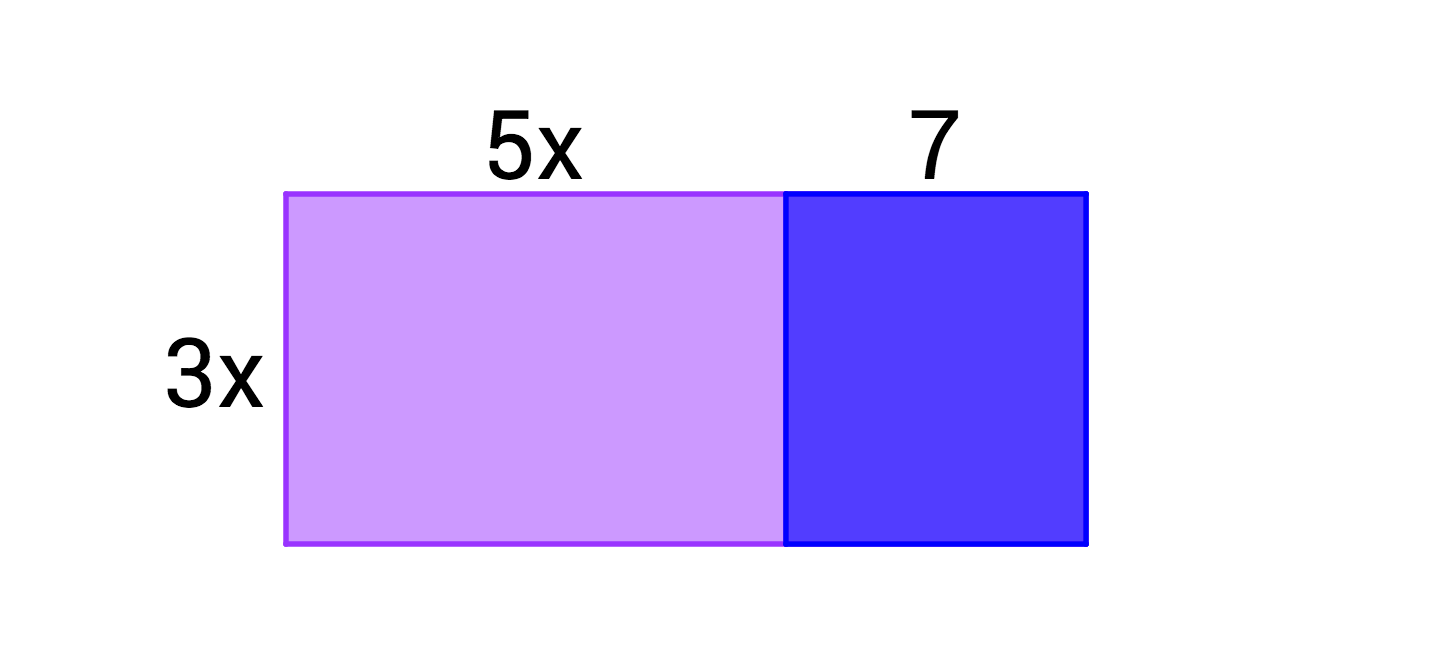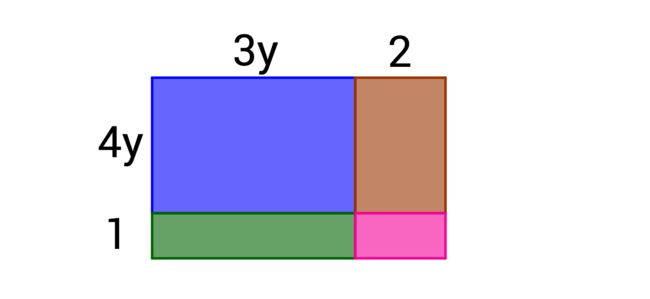Algebra

# Polynomial Arithmetic WarmupWhich expression could not represent the perimeter of the polygon?Which expression could represent the area of the figure?

Which expression could $\color{#D61F06} { \text{not} }$ represent the area of the figure shown?Which expression is $\color{#D61F06} {\text{not}}$ equivalent to $x^{20} - 16x^{64}$ ?

Which statement best describes this equation?

$(f + g)^2 = f^2 + g^2$

×